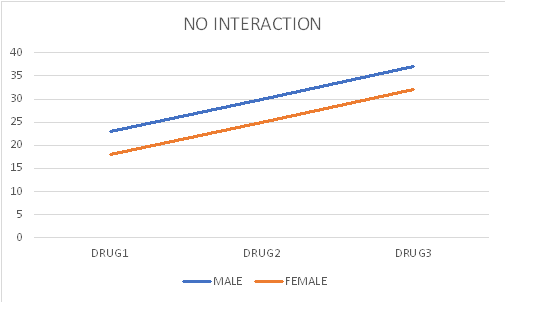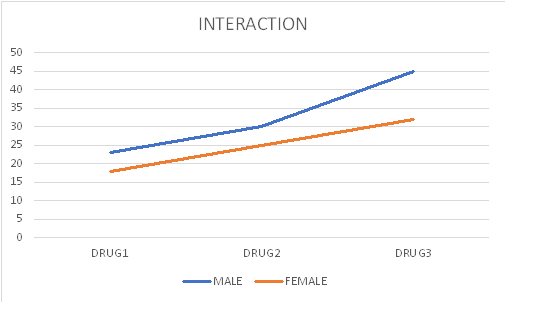# 2 x 3 factorial Analysis of Variance ANOVA- formula and example

### 2 x 3 factorial Analysis of Variance ANOVA- formula and example

#### The layout of a 2x3 factorial ANOVA

B1B2 B3

A1
A1B1
Subject 1
Subject 2
Subject 3
Subject 4
Subject 5
A1B2
Subject 6
Subject 7
Subject 8
Subject 9
Subject 10
A1B3
Subject 11
Subject 12
Subject 13
Subject 14
Subject 15

A2
A2B1
Subject 16
Subject 17
Subject 18
Subject 19
Subject 20
A2B2
Subject 21
Subject 22
Subject 23
Subject 24
Subject 25
A2B3
Subject 26
Subject 27
Subject 28
Subject 29
Subject 30

In this example of a factorial design, we have a 2x3 (we read this as "a two by three") factorial. Two by three, meaning two factors: A and B. "two" meaning two levels for factor A. "three" meaning three levels for B. In another case of a 3x2 factorial design we have two factors, A and B, factor A three levels, factor B two levels.

#### FORMAT OF 2x3 FACTORIAL ANOVA SUMMARY TABLE

Source SS df MS F p
Berween A
Between B
AxB (interaction)
Within
Total

#### Interaction -factorial designs

Note the term "Interaction" in the ANOVA summary table of the factorial design What is interaction? The best way to grasp the concept of interaction is to graph it.### ANOVA 2x3 factorial -practice example

An experimenter wanted to test the effect of two drugs on emotionality of male and female teenagers. He randomly selected 15 male and 15 female teenagers and randomly assigned them to 6 groups: Group 1, Group 2, Group 3, Group 4, Group 5, Group 6. five subjects in each group as shown in the following table.

Drug 1
B1
Drug 2,
B2
Drug 3,
B3

Male
A1
A1B1 Group1

Subject 1
Subject 2
Subject 3
Subject 4
Subject 5
A1B2 Group2

Subject 6
Subject 7
Subject 8
Subject 9
Subject 10
A1B3 Group3

Subject 11
Subject 12
Subject 13
Subject 14
Subject 15

Female
A2
A2B1 Group3

Subject 16
Subject 17
Subject 18
Subject 19
Subject 20
A2B2 Group4

Subject 21
Subject 22
Subject 23
Subject 24
Subject 25
A2B3 Group2

Subject 26
Subject 27
Subject 28
Subject 29
Subject 30

The data are presented on the table below. The scores are the values recorded on a device measuring galvanic skin response, a measure of emotionality. Higher values indicate stronger emotion.

B1B2 B3

A1
A1B1
11
11
13
12
10
A1B2
17
18
17
16
17
A1B3
27
25
24
26
27

A2
A2B1
15
14
14
16
15
A2B2
20
19
18
20
18
A2B3
30
29
29
28
31
.

2x3 FACTORIAL ANOVA SUMMARY TABLE
Source SS df MS F p
Berween A 67.5 1 67.5 62.31 <.0001
Between B 1088.07 2 544.04 502.19 <.0001
AxB (interaction) 3.8 2 1.9 1.75 0.1952
Within 26 24 1.08
Total 1185.37 29

After we calculate the F, we go to the F table to find the required F value for A, B, and AxB (interaction). Because,( remember?) the F ratio is A over within, B over within, AxB over within, we enter the F table with
df of A and df within , which is 1 and 24.
also B and df within, which is 2 and 24
and lastly AxB wihin., which is 2 and 24. We first choose the F tanle at 0.05 level of significance.

Factor A: The F at df 1 and 24 is 4.25. In the summary table we see that the F for factor A (rows) is 62.31, This is greater than 4.25, so we conclude that here we have significance at the 0.05 level of significance; we say p<0.05, p less than 0.05. It has been accepted among scientists that at the 0.05 level we are allowed to say that we have significance, that the finding of our experiment is reliable.

Next we look at factor B. We enter the F table with df 2 and 24 and find F=3.50. This is less than the F of our summary table 502.19, therefore we conclude that we have significance at the 0.05 level. We formally express this as follows: p<0.05.

Next we look at AxB. We enter the F table with df 2 and 24 and find F=3.50.. This is greater than the F at the summary table value of 1.75 so we conclude that here we do not have significance. We formally express this as follows: p>0.05.

Step by step calculation of 2x3 ANOVA factorial The goal of our calculations in ANOVA is to compute the F ratio, The F ratio is MS between over MS within. Mean Square is the mean of the squared deviations (differences) of each score from the mean. These are very simple calculations involving high school mathematics. Simple as they are, they are very important concepts in data analysis and beyond, that is science in general. You will never need to perform these calculations. There are many free Statistics calculators online. However, for the purpose of developing the concepts of ANOVA here are the steps:

1. Calculate the mean of each group.
2. Subtract each score from the mean.
3. Square each difference
4. Add these squared differences. font red This is the Sum of Squares, the SS on the ANOVA summary table.)
5. calculate the degrees of freedom df (number of scores that went into the calculation of the mean minus 1)
6 Divide the SS by the df. Voila! this the MS.
7. The last step is to calculate the F. Divide MS by the MS of the error term (which is the MS within but may be something else depending on which ANOVA design you have. )

The F ratio, as all ratios, compare two things. For example the ratio 8/4 compares 8 to 4 and finds that 8 is two times greater than 4..

Understanding the 2x2 factorial ANOVA summary table

A
Looking at the layout tables above, we see that factor A is gender. Factor B is drug, Our calculations gave a p value <0.05 meaning that factor A, gender, gave a significant difference. In other words, there is a difference in emotionality between male and female

B
.Looking at the layout tables above, we see that factor B is Drug. Our calculations gave a p value <0.05, meaning that factor B, drug, gave a significant difference. In other words, there is a difference in emotionality between subjects that received drug 1 as compared to subjects that received drug 2.

AxB
This is the interaction term. Definition of the interaction. What is interaction in factorial designs? Interaction is present if one level of one factor has a disproportionate effect on one level of the other factor.

Rate it: